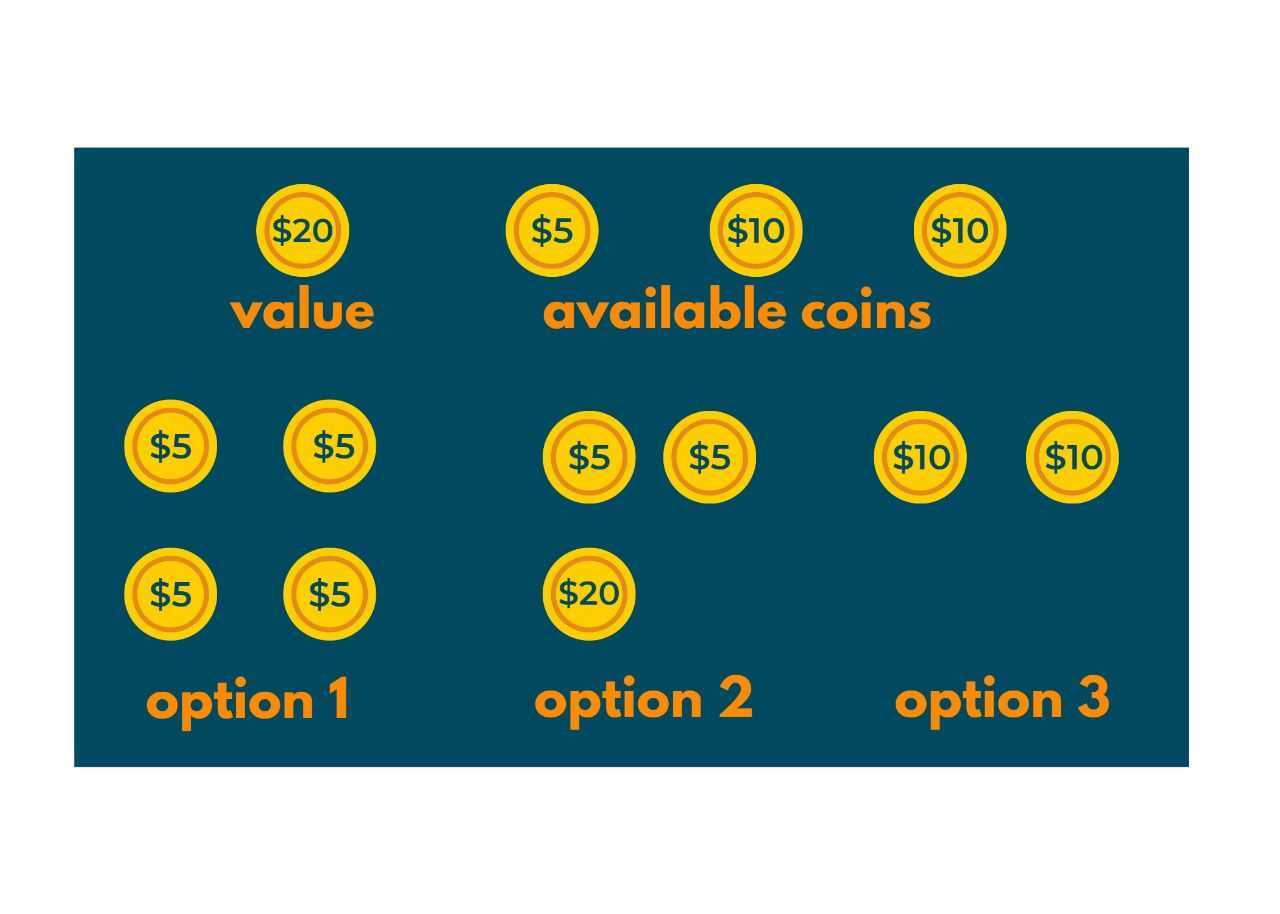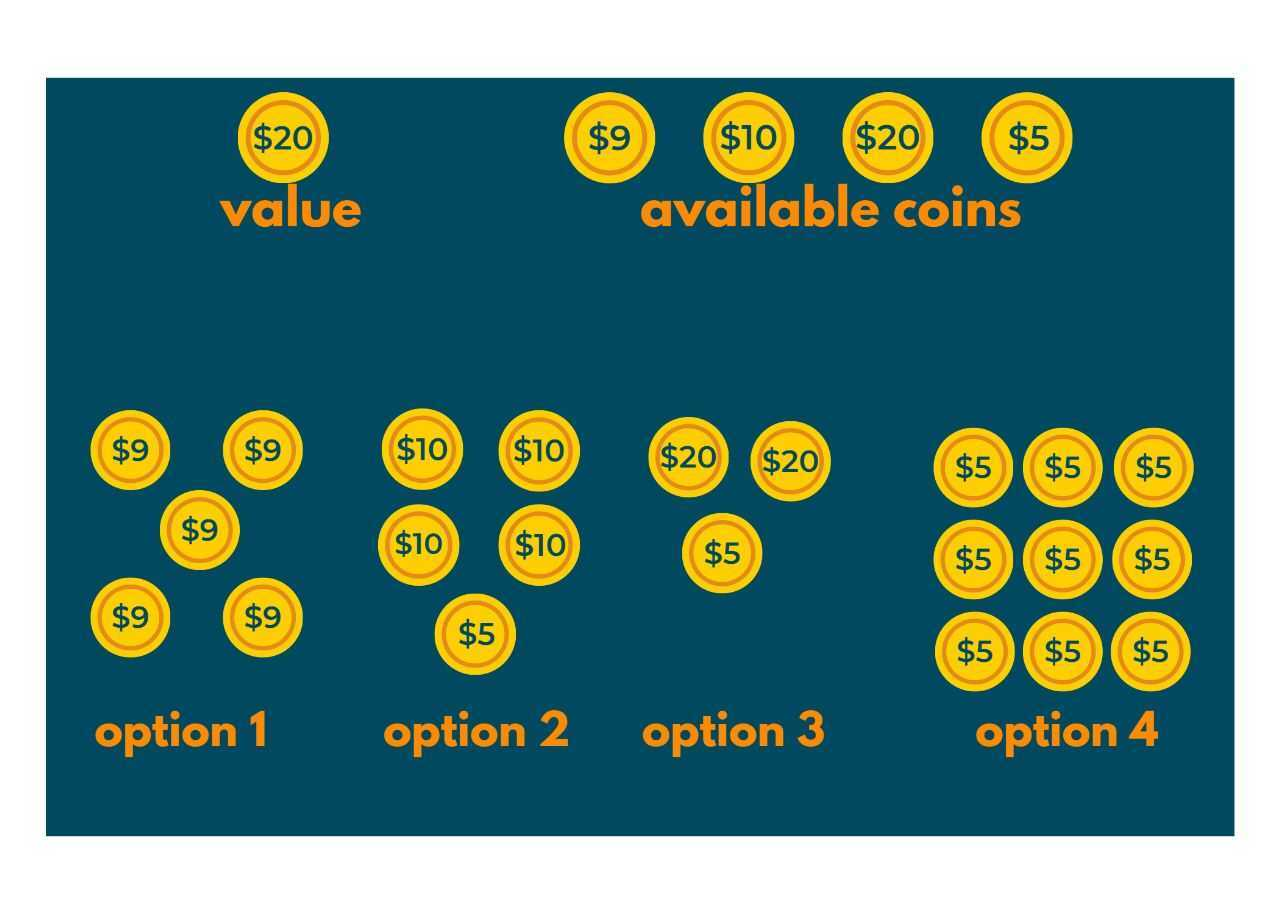# Minimum Coin Change Problem & 2 Solutions (Recursion & DP)

DSA is not a piece of cake! There are endless amounts of questions each getting solved with a different approach using the same Data Structure. Hence, we bring to you another amazing tech blog on a question that can be solved with both recursion as well as dynamic programming.

The Minimum Coin Change problem is actually a variation of the problem where you find whether a change of the given amount exists or not. In this tech blog, we will cover all the details, intuition, and approaches to solving this question. We have provided the solution in both C++ as well Java. Let's get the fun started!

## Minimum Coin Change Problem

Here is the problem statement: You are given a value 'V' and have a limitless supply of given coins. The value of coins is given in an array. You have to find out the minimum number of coins used to convert the value 'V' into a smaller division or change. If there is no possible way, return -1.

### Example 1

Input: V=20, coins[]={5,10,10}

Output: 2

Explanation: We see that value of 20 is required. Now, on traversing the coins array, we have the following options:

1. 4 coins of \$5 each, ∴Total Coins=4
2. 2 coins of \$5 & 1 coin of \$10, ∴Total Coins=3
3. 2 coins of \$10, ∴Total Coins=2

Since 2 coins are the minimum, we get our result =2. You can also take a look at the following image.### Example 2

Input: V=45, coins[]={9, 10, 20, 5}

Output: 3

Explanation: After carefully observing the given values of change coins, we have the following options:

1. 5 coins of \$9 each, ∴Total Coins=5
2. 4 coins of \$10 each & 1 coin of \$5, ∴Total Coins=5
3. 2 coins of \$20 & 1 coin of \$5, ∴Total Coins=3
4. 9 coins of \$5 each, Total Coins=9

Out of the above options, the minimum number of coins is of 3rd option, that is, 3. Hence, the result. Take a look at the following image.### Understanding the Problem

If you have followed the blog step-by-step from the top till now, we can guarantee that you must have a good understanding of what we are actually doing with this question. If you still did not get it, here you go. So, in this question, you are given a value and an array of certain positive integers.

What you need to do is to find the easiest way with which you can exchange the bigger value amount with the smaller coins you have. You may again visit the examples given above. If there are more than 1 way, then the minimum valued one is your answer. If you have traversed through the whole array and still did not get a valid solution, then you need to return a -1 as the answer won't exist in that case.

## Solving Minimum Coin Change Problem

The 2 best methods to solve this problem are using recursion and dynamic programming.:

### Method 01) Using Recursion

In this method, we use recursion to solve this problem.

We first take the base case as to whether the value of V exists or not. It is obvious that if value V=0, then change with the help of coins[] won't be possible. Now, moving forward we take the initial result to be equal to the maximum integer using the in-built function INT_MAX. This function is generally taken when we need to find the minimum result or the smallest value.

Now, we traverse through the array value only if the value of the array element is less than V. Now using the below recursion function we find the answer and return it to the main function.

```minimumCoins(coins[0..size-1], V) = min{1 + minimumCoins(V-coin[i])}
where, i goes from 0 to size-1 and coin[i] <= V```

### Code Implementation in C++

```#include<bits/stdc++.h>
using namespace std;

// size is the length of coins array
int minimumCoins(int coins[], int size, int V)
{
// base case that is, if value is equal to 0, then
if (V == 0)    return 0;
// Initialize result
int res = INT_MAX;
// Try every coin that has smaller value than V
for (int i=0; i<size; i++)
{
if (coins[i] <= V)
{
int sub_res = minCoins(coins, m, V-coins[i]);
if (sub_res != INT_MAX && sub_res + 1 < res)
res = sub_res + 1;
}
}
return res;
}

int main()
{
int coins[] = {9, 6, 5, 1};
int size = sizeof(coins)/sizeof(coins);
int V = 11;
cout << "Minimum coins required is "
<< minimumCoins(coins, size, V);
return 0;
}
```

### Code Implementation in Java

```class Solution
{
// size is the length of array
static int minimumCoins(int coins[], int size, int V)
{
// if there are no coins 	if (V == 0)            return 0;

// Initializing the result with maximum value since minimum is required
int res = Integer.MAX_VALUE;
// Trying every coin that has value less than given V
for (int i=0; i<size; i++)
{
if (coins[i] <= V)
{
int result = minCoins(coins, size, V-coins[i]);
if (result != Integer.MAX_VALUE && result + 1 < res)
res = result + 1;
}
}
return res;
}//main function
public static void main(String args[])
{
int coins[] = {9, 6, 5, 1};
int size = coins.length;
int V = 11;
System.out.println("Minimum coins required is "+ minimumCoins(coins, size, V) );
}
}
```

### Output:

```Minimum coins required is 2
```

### Method 02) Using Dynamic Programming

The method of solving this question using Recursion will work perfectly fine, but what if, your interviewer asks you to optimize it further? Don't worry we got you covered! The time taken by the previous method was exponential so, we will use Dynamic Programming to bring its complexity down further.

In this method, we create a table that will be storing the minimum number of coins required. But, in the beginning, we will initialize it with the maximum number, using the in-built function INT_MAX. Why did we do it? Quite simple! We initialized all the table elements as infinite so that we can get the minimum result in the table created.

Using the below code, we compute the minimum number of coins for the given value V and return it as the answer. Study the below code carefully!

### Code Implementation in C++

```#include <bits/stdc++.h>
using namespace std;

// size is length of coins[] array
int minimumCoins(int coins[], int size, int V)
{
// table[i] will store the result(minimum number of coins)
int table[V + 1];

//Just an edge case if the table element is 0
table = 0;

// Initializing all table elements as infinite using INT_MAX
for (int i = 1; i <= V; i++)
table[i] = INT_MAX;

// Computing minimum values of coins for all array elements
for (int i = 1; i <= V; i++) {
for (int j = 0; j < size; j++)
if (coins[j] <= i) {
int sub_res = table[i - coins[j]];
if (sub_res != INT_MAX && sub_res + 1 < table[i])
table[i] = sub_res + 1;
}
}

if (table[V] == INT_MAX)
return -1;
return table[V];
}

// Driver program to test above function
int main()
{
int coins[] = {9,6,5,1 };
int size= sizeof(coins) / sizeof(coins);
int V = 11;
cout << "Minimum coins required is "
<< minimumCoins(coins, size, V);
return 0;
}
```

### Code Implementation in Java

```import java.io.*;
class Solution
{
// size is the length of coins[] array
static int minimumCoins(int coins[], int size, int V)
{
// table[i] will store minimum value of coins
int table[] = new int[V + 1];

//esge case if table elements are zero
table = 0;

// Initializing all table values as infinite
for (int i = 1; i <= V; i++)
table[i] = Integer.MAX_VALUE;
for (int i = 1; i <= V; i++)
{
for (int j = 0; j < size; j++)
if (coins[j] <= i)
{
int sub_res = table[i - coins[j]];
if (sub_res != Integer.MAX_VALUE
&& sub_res + 1 < table[i])
table[i] = sub_res + 1;
}

}

if(table[V]==Integer.MAX_VALUE)
return -1;

return table[V];

}

public static void main(String[] args)
{
int coins[] = {9,6,5,1};
int size = coins.length;
int V = 11;
System.out.println ( "Minimum coins required is "
+ minimumCoins(coins, size, V));
}
}
```

Output:

```Minimum coins required is 2
```

## Complexity Analysis of Both Methods

Here are the time complexities for both solutions to the problem:

 S.No. Method Name Time Complexity Space Complexity 1. Using Recursion exponential O(n) 2. Using Dynamic Programming O(size*V) O(V)

## Conclusion

So, in this tech blog, we understood the problem of Minimum Coins with 2 methods. You need to understand that both methods work perfectly fine, it's just a matter of choice to use Recursion or Dynamic Programming. Although, to land your dream job you should learn both these concepts by heart and solve as many questions as possible.

FavTutor will continue to bring such great tech blogs clearing your doubts regarding advanced concepts, so Stay Tuned!

### FavTutor - 24x7 Live Coding Help from Expert Tutors!